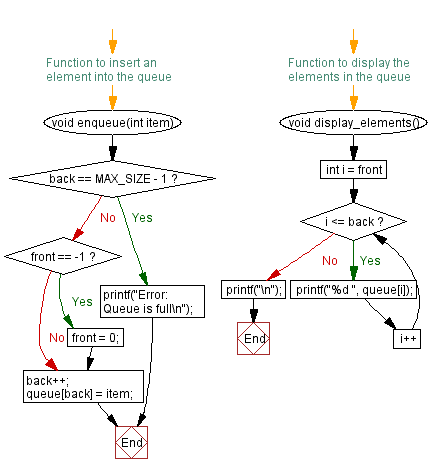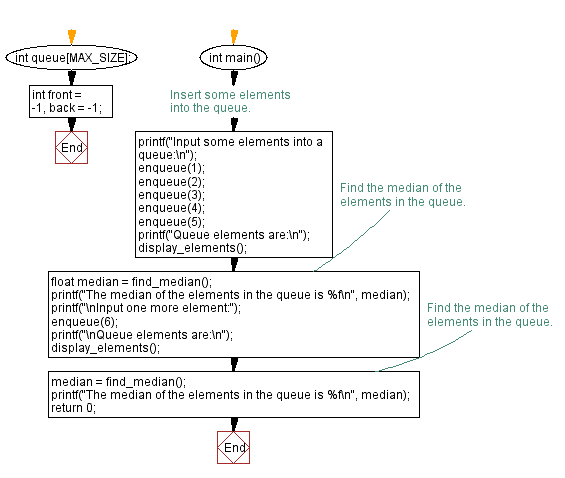﻿ C - Find the median of the elements in a queue

# C Exercises: Find the median of the elements in a queue

## C Queue: Exercise-13 with Solution

Write a C program to find the median of the elements in a queue.

From Wikipedia,
In statistics and probability theory, the median is the value separating the higher half from the lower half of a data sample, a population, or a probability distribution.
The median of a finite list of numbers is the "middle" number, when those numbers are listed in order from smallest to greatest.
If the data set has an odd number of observations, the middle one is selected. For example, the following list of seven numbers,
1, 3, 3, 6, 7, 8, 9
has the median of 6, which is the fourth value.
If the data set has an even number of observations, there is no distinct middle value and the median is usually defined to be the arithmetic mean of the two middle values. For example, this data set of 8 numbers1, 2, 3, 4, 5, 6, 8, 9 has a median value of 4.5, that is (4+5)/2 . (In more technical terms, this interprets the median as the fully trimmed mid-range).

Sample Solution:

C Code:

``````#include <stdio.h>

#define MAX_SIZE 100
int queue[MAX_SIZE];
int front = -1, back = -1;
// Function to insert an element into the queue
void enqueue(int item) {
if (back == MAX_SIZE - 1) {
printf("Error: Queue is full\n");
return;
}
if (front == -1) {
front = 0;
}
back++;
queue[back] = item;
}

// Function to remove an element from a queue
int dequeue() {
if (front == -1 || front > back) {
printf("Error: Queue is empty\n");
return -1;
}
int item = queue[front];
front++;
return item;
}

// Function to find the median of the elements in the queue
float find_median() {
// First, make a copy of the queue
int n = back - front + 1;
int temp[n];
for (int i = 0; i < n; i++) {
temp[i] = queue[front + i];
}
// Then, sort the copy in ascending order
for (int i = 0; i < n; i++) {
for (int j = i + 1; j < n; j++) {
if (temp[j] < temp[i]) {
int t = temp[i];
temp[i] = temp[j];
temp[j] = t;
}
}
}
// Calculate the median based on the sorted copy
if (n % 2 == 0) {
int m1 = temp[n/2 - 1];
int m2 = temp[n/2];
return (float)(m1 + m2) / 2;
} else {
int m = temp[n/2];
return (float)m;
}
}

// Function to display the elements in the queue
void display_elements() {
for (int i = front; i <= back; i++) {
printf("%d ", queue[i]);
}
printf("\n");
}

int main() {
// Insert some elements into the queue.
printf("Input some elements into a queue:\n");
enqueue(1);
enqueue(2);
enqueue(3);
enqueue(4);
enqueue(5);
printf("Queue elements are:\n");
display_elements();
// Find the median of the elements in the queue.
float median = find_median();
printf("The median of the elements in the queue is %f\n", median);
printf("\nInput one more element:");
enqueue(6);
printf("\nQueue elements are:\n");
display_elements();
// Find the median of the elements in the queue.
median = find_median();
printf("The median of the elements in the queue is %f\n", median);
return 0;
}
```
```

Sample Output:

```Input some elements into a queue:
Queue elements are:
1 2 3 4 5
The median of the elements in the queue is 3.000000

Input one more element:
Queue elements are:
1 2 3 4 5 6
The median of the elements in the queue is 3.500000
```

Flowchart:C Programming Code Editor:

Have another way to solve this solution? Contribute your code (and comments) through Disqus.

Previous: Sort a queue in ascending order.

What is the difficulty level of this exercise?

Test your Programming skills with w3resource's quiz.

﻿

## C Programming: Tips of the Day

C Programming - How do you pass a function as a parameter in C?

Declaration

A prototype for a function which takes a function parameter looks like the following:

```void func ( void (*f)(int) );
```

This states that the parameter f will be a pointer to a function which has a void return type and which takes a single int parameter. The following function (print) is an example of a function which could be passed to func as a parameter because it is the proper type:

```void print ( int x ) {
printf("%d\n", x);
}
```

Function Call

When calling a function with a function parameter, the value passed must be a pointer to a function. Use the function's name (without parentheses) for this:

```func(print);
```

would call func, passing the print function to it.

Function Body

As with any parameter, func can now use the parameter's name in the function body to access the value of the parameter. Let's say that func will apply the function it is passed to the numbers 0-4. Consider, first, what the loop would look like to call print directly:

```for ( int ctr = 0 ; ctr < 5 ; ctr++ ) {
print(ctr);
}
```

Since func's parameter declaration says that f is the name for a pointer to the desired function, we recall first that if f is a pointer then *f is the thing that f points to (i.e. the function print in this case). As a result, just replace every occurrence of print in the loop above with *f:

```void func ( void (*f)(int) ) {
for ( int ctr = 0 ; ctr < 5 ; ctr++ ) {
(*f)(ctr);
}
}
```

Ref : https://bit.ly/3skw9Um